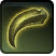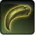# SchlagConditions

## Used by

Used by
• Class 16141176590901306922

## Related effects, buffs and debuffs

Please click on an effect below to view its details.

• [0s] [not displayed]Effect #1

 Slot: Debuff Duration: 0 Sek. Tick rate: does not tick # occurrences: 0
• On Apply
• [target override]

Perform the following actions:

• Add effect #2 to TARGET from TARGET
• [3.6s]Schlag

 Slot: Debuff Duration: 3.6 Sek. Tick rate: does not tick # occurrences: 1 Conditions: Can only by called by other effects
• On Apply

Perform the following actions:

• Play appearance epp . operation . iokath . boss . izax . izax . p5_swipe_left_fast, dependent on calling effect
• Unknown (183)
- Unknown (303) = (bool) true
- Unknown (306) = (bool) false
- Unknown (430) = (bool) false
- Unknown (310) = (string) aoePositionOptionType_Entity
- Unknown (311) = (string) aoeTarget_Caster
- Unknown (313) = (string) aoeScalarType_LiteralValue
- Unknown (317) = (string) aoeScalarType_LiteralValue
- Unknown (321) = (string) aoeScalarType_LiteralValue
- Unknown (325) = (string) aoeOrientationOptionType_EntityFacing
- Unknown (326) = (string) aoeTarget_Caster
- Unknown (344) = (string) aoeCone
- Unknown (349) = (string) aoeTargetCondition_IsAlive;aoeTargetCondition_IsEnemy[aoeParameter_Enemyof:aoeConditionTarget_Caster]
- Unknown (353) = (string) aoeScalarType_LiteralValue
- Unknown (354) = (string) aoeScalarType_LiteralValue
- Unknown (355) = (string) aoeScalarType_LiteralValue
- Unknown (375) = (string) aoeScalarType_LiteralValue
- Unknown (384) = (string)
- Unknown (386) = (string) aoeAbilityCaster_Caster
- Unknown (387) = (string) aoeAbilityTarget_Target
- Unknown (405) = (string) aoeScalarType_LiteralValue
- Unknown (431) = (string) aoeRotation_None
- Ability Spec = (int) 16140967419466099653
- Unknown (307) = (int) 1
- Unknown (308) = (int) 1
- Tick Rate = (float) 0.5
- Unknown (304) = (float) 1.25
- Unknown (305) = (float) 1.25
- Unknown (314) = (float) 0
- Unknown (318) = (float) 0
- Unknown (322) = (float) 0
- Unknown (362) = (float) 0
- Unknown (363) = (float) 0
- Unknown (364) = (float) 0
- Unknown (376) = (float) 2
- Unknown (390) = (float) 0.9
- Unknown (398) = (float) 0.75
- Unknown (406) = (float) 60
• Unknown (183)
- Unknown (303) = (bool) true
- Unknown (306) = (bool) false
- Unknown (430) = (bool) false
- Unknown (310) = (string) aoePositionOptionType_Entity
- Unknown (311) = (string) aoeTarget_Caster
- Unknown (313) = (string) aoeScalarType_LiteralValue
- Unknown (317) = (string) aoeScalarType_LiteralValue
- Unknown (321) = (string) aoeScalarType_LiteralValue
- Unknown (325) = (string) aoeOrientationOptionType_EntityFacing
- Unknown (326) = (string) aoeTarget_Caster
- Unknown (344) = (string) aoeCone
- Unknown (349) = (string) aoeTargetCondition_IsAlive;aoeTargetCondition_IsEnemy[aoeParameter_Enemyof:aoeConditionTarget_Caster]
- Unknown (353) = (string) aoeScalarType_LiteralValue
- Unknown (354) = (string) aoeScalarType_LiteralValue
- Unknown (355) = (string) aoeScalarType_LiteralValue
- Unknown (375) = (string) aoeScalarType_LiteralValue
- Unknown (384) = (string)
- Unknown (386) = (string) aoeAbilityCaster_Caster
- Unknown (387) = (string) aoeAbilityTarget_Target
- Unknown (405) = (string) aoeScalarType_LiteralValue
- Unknown (431) = (string) aoeRotation_None
- Ability Spec = (int) 16140967419466099653
- Unknown (307) = (int) 1
- Unknown (308) = (int) 1
- Tick Rate = (float) 0.5
- Unknown (304) = (float) 1.25
- Unknown (305) = (float) 1.25
- Unknown (314) = (float) 0
- Unknown (318) = (float) 0
- Unknown (322) = (float) 0
- Unknown (362) = (float) 0
- Unknown (363) = (float) -70
- Unknown (364) = (float) 0
- Unknown (376) = (float) 2
- Unknown (390) = (float) 0.9
- Unknown (398) = (float) 0.9
- Unknown (406) = (float) 80